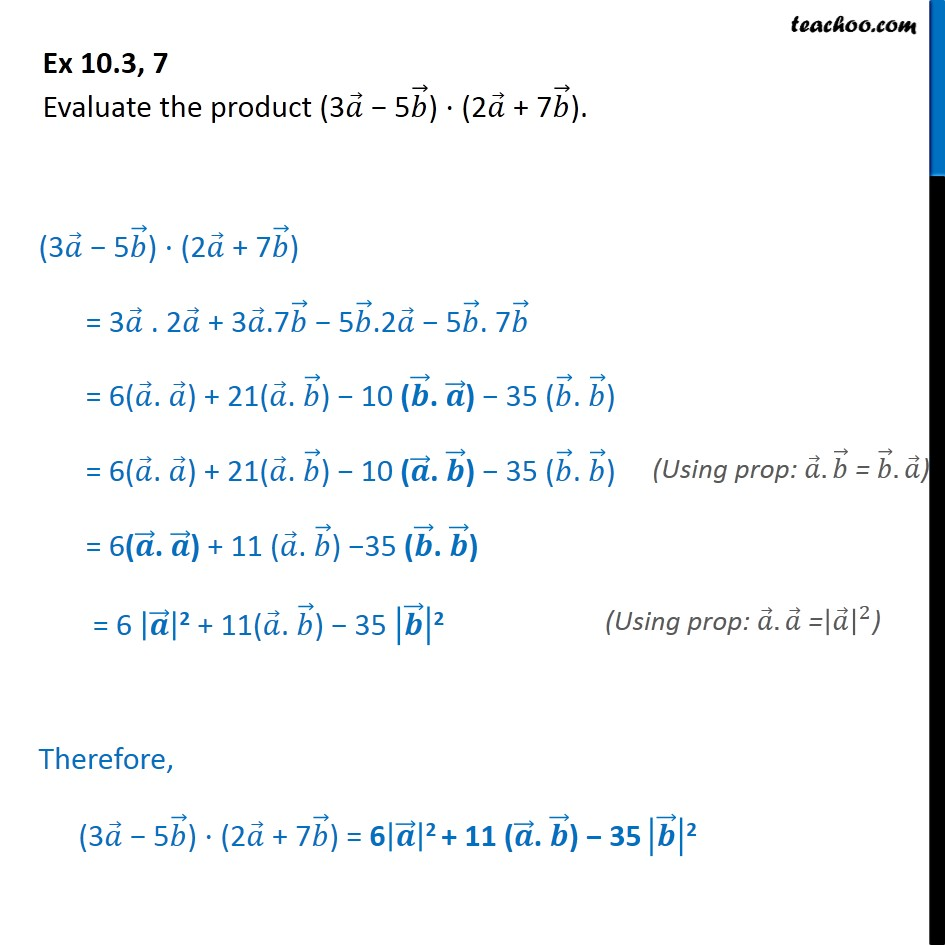Subscribe to our Youtube Channel - https://you.tube/teachoo

1. Chapter 10 Class 12 Vector Algebra
2. Serial order wise
3. Ex 10.3

Transcript

Ex 10.3, 7 Evaluate the product (3 𝑎﷯ − 5 𝑏﷯) ⋅ (2 𝑎﷯ + 7 𝑏﷯). (3 𝑎﷯ − 5 𝑏﷯) ⋅ (2 𝑎﷯ + 7 𝑏﷯) = 3 𝑎﷯ . 2 𝑎﷯ + 3 𝑎﷯.7 𝑏﷯ − 5 𝑏﷯.2 𝑎﷯ − 5 𝑏﷯. 7 𝑏﷯ = 6( 𝑎﷯. 𝑎﷯) + 21( 𝑎﷯. 𝑏﷯) − 10 ( 𝒃﷯. 𝒂﷯) − 35 ( 𝑏﷯. 𝑏﷯) = 6( 𝑎﷯. 𝑎﷯) + 21( 𝑎﷯. 𝑏﷯) − 10 ( 𝒂﷯. 𝒃﷯) − 35 ( 𝑏﷯. 𝑏﷯) = 6( 𝒂﷯. 𝒂﷯) + 11 ( 𝑎﷯. 𝑏﷯) −35 ( 𝒃﷯. 𝒃﷯) = 6 𝒂﷯﷯2 + 11( 𝑎﷯. 𝑏﷯) − 35 𝒃﷯﷯2 Therefore, (3 𝑎﷯ − 5 𝑏﷯) ⋅ (2 𝑎﷯ + 7 𝑏﷯) = 6 𝒂﷯﷯2 + 11 ( 𝒂﷯. 𝒃﷯) − 35 𝒃﷯﷯2

Ex 10.3